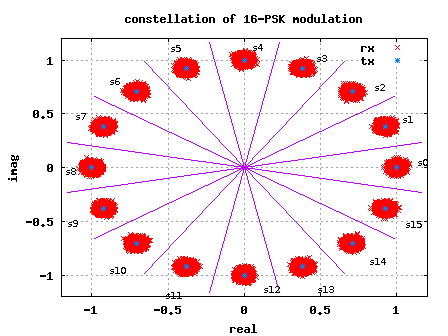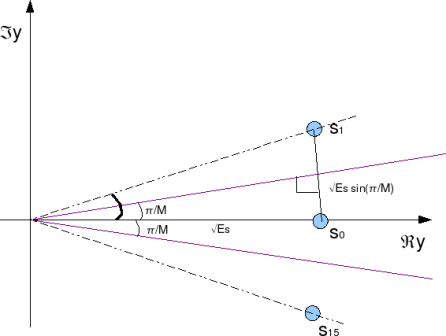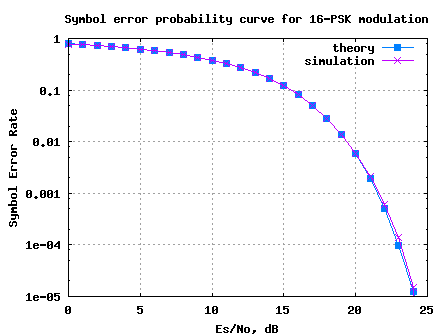- DSP log - http://www.dsplog.com -

Symbol Error Rate for 16PSK

Posted By Krishna Sankar On March 18, 2008 @ 5:10 am In Modulation | 31 Comments

In this post, let us try to derive the symbol error rate for 16-PSK (16-Phase Shift Keying) modulation.

Consider a general M-PSK modulation, where the alphabets,

are used.

(Refer example 5-38 in [DIG-COMM-BARRY-LEE-MESSERSCHMITT])Figure: 16-PSK constellation plot

## Deriving the symbol error rate

Let us the consider the symbol on the real axis, i.e

.

Where the additive noise follows the Gaussian probability distribution function,

with and .

The conditional probability distribution function (PDF) of received symbol given was transmitted is:

.

As can be seen from the figure above, due to the addition of noise, the transmitted symbol gets spreaded. However, if the received symbol is present with in the boundary defined by the magenta lines, then the symbol will be demodulated correctly.

To derive the symbol error rate, the objective is to find the probability that the phase of the received symbol lies within this boundary defined by the magenta lines i.e. from to .

For simplifying the derivation, let us make the following assumptions:

(a) The signal to noise ratio, is reasonably high.

For a reasonably high value of , then the real part of the received symbol is not afected by noise i.e.,

and

the imaginary part of the received symbol is equal to noise, i.e.
.

(b) The value of M is reasonably high (typically M >4 suffice)

For a reasonably high value of M, the constellation points are closely spaced. Given so, the distance of the constellation point to the magenta line can be approximated as .Figure: Distance between constellation points

Given the above two assumptions, it can be observed that the symbol will be decoded incorrectly, if the imaginary component of received symbol isgreater than . The probability of being greater than is,

.

Changing the variable to ,

.

Note: The complementary error function, .

Similarly, the symbol will be decoded incorrectly, if the imaginary component of received symbol is less than . The probability of being less than is,

.

The total probability of error given was transmittd is,

.

Total symbol error rate

The symbol will be in error, if atleast one of the symbol gets decoded incorrectly. Hence the total symbol error rate from M-PSK modulation is,

.

## Simulation model

Simple Matlab/Octave script for simulating transmission and recepetion of an M-PSK modulation is attached. It can be observed that the simulated symbol error rate compares well with the theoretical symbol error rate.Figure: Symbol Error rate curve for 16PSK modulation

Hope this helps.
Krishna

References

[DIG-COMM-BARRY-LEE-MESSERSCHMITT]Digital Communication: Third Edition, by John R. Barry, Edward A. Lee, David G. Messerschmitt